# Introduction

This article is a tutorial of the code implemented in the repository stereo-dense-reconstruction. The theory is not covered in detail, so some basic reading on the topic is suggested before one delves into the code. This ROS package computes dense disparity maps using LIBELAS and converts them into 3D point clouds. The method for generation of disparity maps can be changed based on user preferences. I had written an article on generating dense disparity maps using ORB descriptors earlier. That method can be implemented real time by using optimized code and parallelization.

An overview of the working code can be seen in the above video. Generation of dense disparity maps and the resulting point clouds is shown. The visualizations are shown in rviz. Towards the end of the video, point cloud transformation is demonstrated using rqt_reconfigure.

# Stereo Calibration

A calibrated pair of stereo cameras is required for dense reconstruction. The calibration file is stored as a YAML file containing the following information about the stereo cameras:

Name Description
K1 Intrinsic matrix of left camera
K2 Intrinsic matrix of right camera
D1 Distortion coefficients of left camera
D2 Distortion coefficients of right camera
R Rotation matrix from left camera to right camera
T Translation vector from left camera to right camera
XR Rotation matrix for point cloud transformation
XT Translation matrix for point cloud transformation

See a sample calibration file. A YAML calibration file can be generated by using this stereo camera calibration tool. For more information see this detailed tutorial on stereo camera calibration.

Although we require only the matrices mentioned in the above table, the sample calibration file contains other matrices found after rectification (e.g., P1, P2, Q etc.). These matrices are found by OpenCV’s stereoRectify function and they depend on the output image resolution of the rectified stereo images. Hence it is better to run stereoRectify online than storing those matrices in a calibration file. This gives us the flexibility to work at different image resolutions.

The use of XR and XT matrices is explained later. For initial values XR can be set as the $3 \times 3$ identity matrix while XT can be set as the $3 \times 1$ zero vector.

# Stereo Rectification

Disparity maps are generated from rectified stereo images. The raw stereo images should be published by a topic which publishes messages of the type sensor_msgs/Image. The code for rectification of stereo images is implemented in src/stereo_rectify.cpp.

Declare all the calibration matrices as Mat variables. calib_img_size is the resolution of the images used for stereo calibration. out_img_size is the output resolution of the rectified images. This can be set at our own will. Lower resolution images require less computation time for dense reconstruction. pub_img_left and pub_img_right are the publishers for the rectified images. The output images are published as a sensor_msgs/CompressedImage message. This is helpful while collecting large datasets, so that file size is reduced.

This function undistorts and rectifies the src image into dst. The homographic mappings lmapx, lmapy, rmapx, and rmapy are found from OpenCV’s initUndistortRectifyMap function.

This function computes all the projection matrices and the rectification transformations using the stereoRectify and initUndistortRectifyMap functions respectively.

This callback function takes a raw stereo (left) image as input. Then it undistorts and rectifies the image using the functions defined above and publishes on the rectified image topic using pub_img_left. Similarly imgRightCallback is defined for the right stereo image.

In the main function, the stereo calibration information is read into calib_file. sub_img_left and sub_img_right subscribes to the topics publishing the raw stereo image data. The rectified output images are published to the following topics:

• /camera_left_rect/image_color
• /camera_right_rect/image_color

The ros node can be run as

# Dense 3D Reconstruction

Generating dense 3D reconstructions involve two major steps: (1) computing a disparity map (2) converting the disparity map into a 3D point cloud. The code for dense reconstruction is implemented in src/dense_reconstruction.cpp.

Having time synced stereo images is important for generating accurate disparity maps. To sync the left and right image messages by their header time stamps, ApproximateTime is used. The procedure is implemented in the main function of src/dense_reconstruction.cpp.

This syncs the two rectified image topics, and the callback function imgCallback receives the time synced messages.

## Disparity Maps using LIBELAS

LIBELAS (Efficient Large-Scale Stereo Matching) is a great open source software for generating dense disparity maps. It does not use global optimization techniques and yet produces great results at almost real time. More information about ELAS can be found here.

This function computes the dense disparity map using LIBELAS, and publishes it as a 8-bit grayscale image to the topic /camera_left_rect/disparity_map. The disparity map is constructed with the left image as reference. The parameters for LIBELAS can be changed in the file src/elas/elas.h.

Any method other than LIBELAS can be implemented inside the generateDisparityMap function to generate disparity maps. One can use OpenCV’s StereoBM class as well. The output should be a 8-bit grayscale image.

## 3D Reconstruction

Given a disparity map, a corresponding 3D point cloud can be easily constructed. The Q matrix stored in the calibration file is used for this conversion. The reconstruction is mathematically expressed by the following matrix equation.

$\begin{bmatrix} X \\ Y \\ Z \\ W \\ \end{bmatrix} = \begin{bmatrix} 1 & 0 & 0 & -c_x \\ 0 & 1 & 0 & -c_y \\ 0 & 0 & 0 & f \\ 0 & 0 & -1/T_x & (c_x - c_x')/T_x \\ \end{bmatrix} \begin{bmatrix} x \\ y \\ d(x, y) \\ 1 \end{bmatrix}\\$

where $(X,Y,Z,W)^\top$ denotes the 3D point in homogeneous coordinates. $d(x,y)$ is the disparity of a point $(x,y)$ in the left image. The $4 \times 4$ matrix denotes the Q matrix. This matrix multiplication in homogeneous coordinates is implemented in the publishPointCloud function. It publishes a sensor_msgs/PointCloud message to the topic camera_left_rect/point_cloud. The point cloud generated is in the reference frame of the left camera. Hence a transformation (XR, XT) is applied to transform the point cloud into a different reference frame (as required by the user). The transformation equation is as follows

$P_B = R \times P_A + T$

where $P_A$ is a point in reference frame $A$, $P_B$ is a point in the reference frame $B$. $R$ and $T$ denote the rotation and translation matrices respectively. In this case $R$ = XR and $T$ = XT. Figure 1 shows some visualizations of the resulting point clouds from dense disparity maps.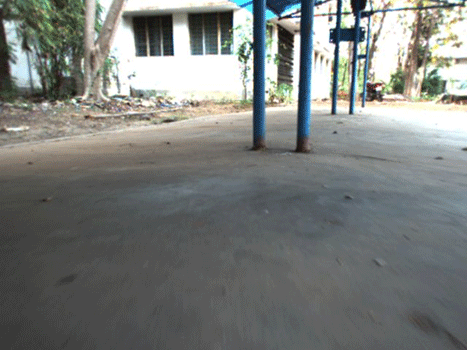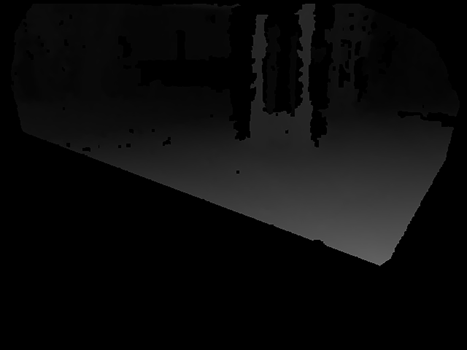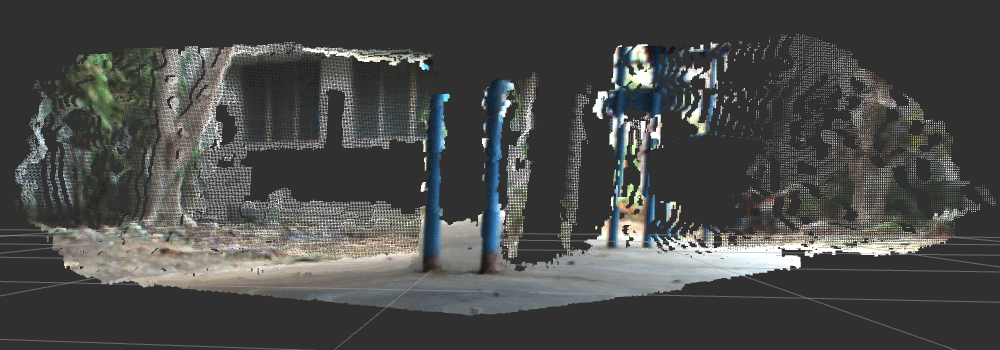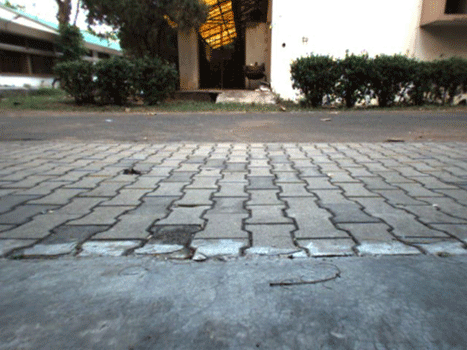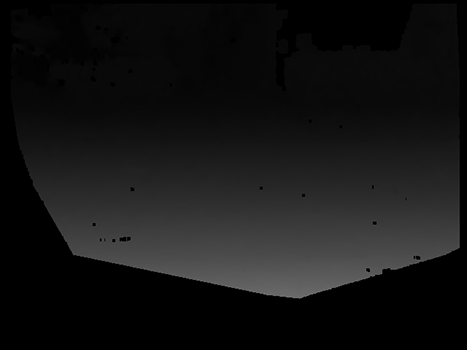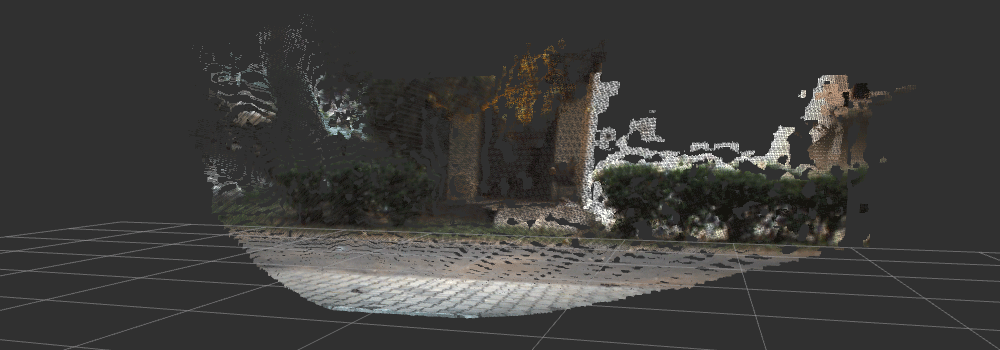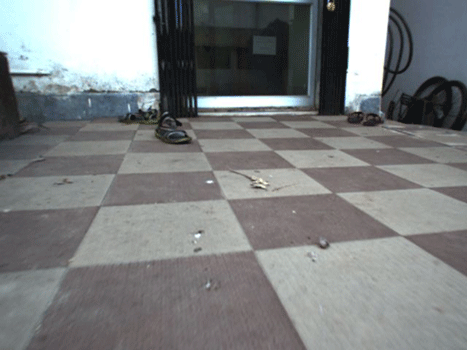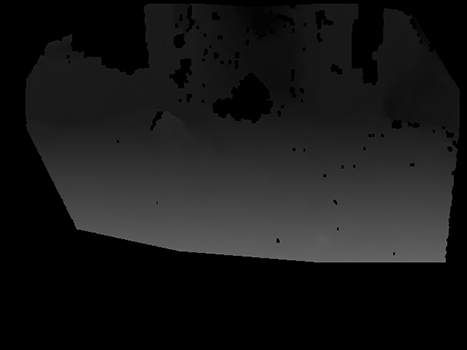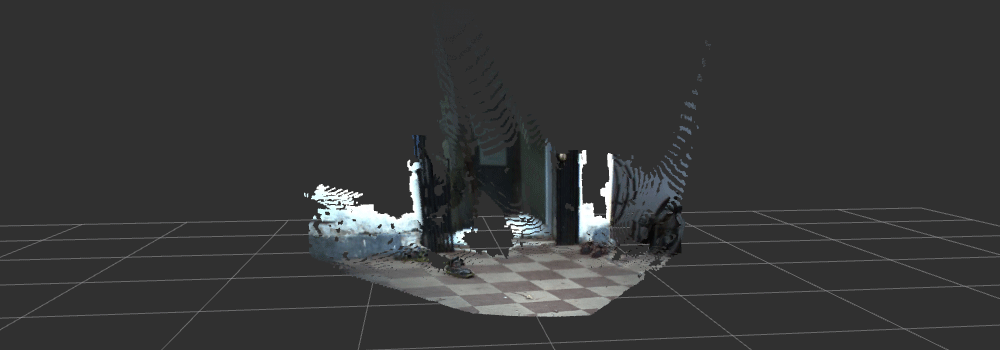Fig. 1: Visualizations of the dense disparity maps (generated by LIBELAS) and the resulting point clouds. The data set was collected by a Point Grey Bumblebee camera onboard a Jackal UGV at IIT Kharagpur. (a) Left Image (b) Disparity Map (c) 3D Point Cloud.

Run the dense reconstruction node as

Visualize the point cloud in rviz

# Point Cloud Transformation

For ground robots with mounted stereo cameras, usually the point clouds are formed in the reference frame of the left camera. Often the point clouds need to be transformed to a different frame e.g., a reference frame with the origin at the centre of rotation of the robot projected on to the ground plane. These transformations are hard to calculate mathematically. Hence it is easier to find these transformations visually, if the geometry of the scene is known.

The transformation from one reference frame to another is represented by the rotation matrix XR and the translation vector XT. XR can be decomposed into Euler angles ($\phi_x, \phi_y, \phi_z$) while XT contains the translation values in the $X,Y,$ and $Z$ direction. A config file cfg/CamToRobotCalibParams.cfg stores these six values. The config file is bound with the dense_reconstruction node using dynamic_reconfigure.

The above piece of code implements dynamic_reconfigure to configure the transformation parameters.

The above two functions are used to compose the matrices XR and XT. composeRotationCamToRobot finds a rotation matrix from three Euler angles by the $ZYX$ convention. composeTranslationCamToRobot returns a $3\times 1$ translation matrix.

Initially, the values of XR and XT in the calibration file were the $3\times 3$ identity matrix and the $3\times 1$ zero matrix. The function debugCamToRobotTransformation(XR, XT) can be used to dynamically change the values of XR and XT with the help of dynamic_reconfigure. If the desired transformation is found, the resulting values of XR and XT can be updated in the calibration file. To modify the transformation values start dynamic_reconfigure by

Refer to the video in the Introduction section to have a better understanding of how the point cloud transformation works. It is shown towards the end of the video.

# Build and Run

Clone the repository stereo-dense-reconstruction in your ros catkin workspace. Build using catkin_make. Further instructions on running each node is given in the README of the repository.